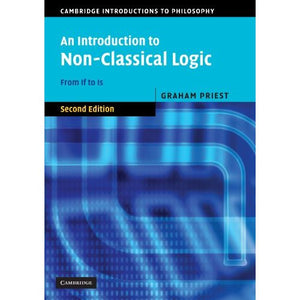># An Introduction to Non-Classical Logic, Second Edition: From If to Is (Cambridge Introductions to Philosophy)

• £16.79
• Save £14

Graham Priest
Cambridge University Press
Edition: 2, 4/10/2008
EAN 9780521670265, ISBN10: 0521670268

Paperback, 646 pages, 24.7 x 17.4 x 3.6 cm
Language: English

This revised and considerably expanded 2nd edition brings together a wide range of topics, including modal, tense, conditional, intuitionist, many-valued, paraconsistent, relevant, and fuzzy logics. Part 1, on propositional logic, is the old Introduction, but contains much new material. Part 2 is entirely new, and covers quantification and identity for all the logics in Part 1. The material is unified by the underlying theme of world semantics. All of the topics are explained clearly using devices such as tableau proofs, and their relation to current philosophical issues and debates are discussed. Students with a basic understanding of classical logic will find this book an invaluable introduction to an area that has become of central importance in both logic and philosophy. It will also interest people working in mathematics and computer science who wish to know about the area.

Preface to the first edition
Preface to the second edition
Mathematical prolegomenon
Part I. Propositional Logic
1. Classical logic and the material conditional
2. Basic modal logic
3. Normal modal logics
4. Non-normal modal logics
strict conditionals
5. Conditional logics
6. Intuitionist logic
7. Many-valued logics
8. First degree entailment
9. Logics with gaps, gluts, and worlds
10. Relevant logics
11. Fuzzy logics
11a. Appendix
many valued modal logics
Postscript
an historical perspective on conditionals
Part II. Qualification and Identity
12. Classical logic
13. Free logic
14. Constant domain modal logics
15. Variable domain modal logics
16. Necessary identity in modal logic
17. Contingent identity in modal logic
18. Non-normal modal logics
19. Conditional logics
20. Intuitionist logic
21. Many-valued logics
22. First degree entailment
23. Logics with gaps, gluts, and worlds
24. Relevant logics
25. Fuzzy logics
Postscript
a methodological coda.

'Priest's Introduction to Non-Classical Logic is my textbook of choice for introducing non-classical logic to undergraduates. It is unique in meeting two almost inconsistent aims. It gives the reader an introduction to a vast range of non-classical logics. No comparable textbook manages to cover modal logics, conditional logics, intuitionistic logic, relevant and paraconsistent logics and fuzzy logic with such clarity and accessibility. Amazingly, it is not merely a catalogue of different logical systems. The distinctive value of this Introduction is that it also tells a coherent story: Priest weaves together these different logics in the one narrative - the search for a logic of conditionals. With the publication of the second volume, this unique combination of breadth and coherence now covers much more ground, and the reader now has an expert guide to much more of the vast field of research in non-classical logics.' Greg Restall, The University of Melbourne